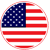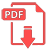# Home | Mathematics | Graphs

## Line Graph

The graph of a linear equation in two variables is a line (that's why they call it linear ).

If you know an equation is linear, you can graph it by finding any two solutions

(x1,y1) and (x2,y2),

plotting these two points, and drawing the line connecting them.English